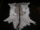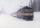Cooks

Four cooks cleaned 5 kg of potatoes for 10 minutes. How many cook would have to work clean 9 kg of potatoes for 12 minutes?

Result

x =  6

Solution:

12x/9 = 4*10/5

60x = 360

x = 6

Calculated by our simple equation calculator.

Leave us a comment of this math problem and its solution (i.e. if it is still somewhat unclear...):Be the first to comment!To solve this verbal math problem are needed these knowledge from mathematics:

Need help calculate sum, simplify or multiply fractions? Try our fraction calculator. Do you solve Diofant problems and looking for a calculator of Diofant integer equations?

Next similar examples:

1. BarterThere is exchange trade on the market. We know that for two sheepskins we get three goat skins. We also know that for six rabbits skins we get four goat skins. How many rabbit's skins we get for four sheepskins?
2. TrainThe train passes part of the line for 95 minutes at speed 75 km/h. What speed would have to go in order to shorten the driving time of 20 minutes?
3. Fractions 4How many 2/3s are in 6?
4. Math classificationIn 3A class are 27 students. One-third got a B in math and the rest got A. How many students received a B in math?
5. PointsGryffindor won 437 points. How many points obtained by each of the faculties if they were split at a ratio of 5: 7: 3: 4?
6. The resultHow many times I decrease the number 1632 to get the result 24?
7. Write 2Write 791 thousandths as fraction in expanded form.
8. AndreAndre, Thomas, and Ivan split 88 postage stamps in a 2:5:4 ratio. How much did Thomas get?
9. Pizza 4Marcus ate half pizza on monday night. He than ate one third of the remaining pizza on Tuesday. Which of the following expressions show how much pizza marcus ate in total?
10. Cake 71/3 of a cake shared with 4 people. What share of the whole cake has each people?
11. Mixed2improperWrite the mixed number as an improper fraction. 166 2/3
12. Product of two fractionsProduct of two fractions is 9 3/5 . If one of the fraction is 9 3/7. Find the other fraction.
13. In fractionsAn ant climbs 2/5 of the pole on the first hour and climbs 1/4 of the pole on the next hour. What part of the pole does the ant climb in two hours?
14. ZdeněkZdeněk picked up 15 l of water from a 100-liter full-water barrel. Write a fraction of what part of Zdeněk's water he picked.
15. CagesHonza had three cages (black, silver, gold) and three animals (guinea pig, rat and puppy). There was one animal in each cage. The golden cage stood to the left of the black cage. The silver cage stood on the right of the guinea pig cage. The rat was in the
16. Addition of Roman numbersAdded together and write as decimal number: LXVII + MLXIV
17. TeacherTeacher Rem bought 360 pieces of cupcakes for the outreach program of their school. 5/9 of the cupcakes were chocolate flavor and 1/4 wete pandan flavor and the rest were a vanilla flavor. How much more pandan flavor cupcakes than vanilla flavor?